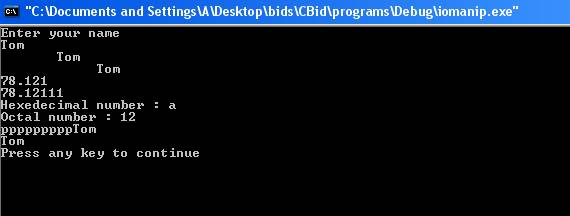iomanip

The iomanip is a parameterized input output manipulator. In order to access manipulators that take parameters the header file <iomanip> is included in the program. Some of the manipulators are:-

• setioflags- It sets the flags controlling the input output stream.

• resetiosflags -  It resets the flags controlling the input output stream.

• setbase(int n) – It sets the output representation of the number to octal, decimal or hexadecimal corresponding to the argument n which is 8 in case of octal, 10 for decimal and 16 for hexadecimal. Any other value will not change the base.

• setfill ( char c) – It sets the fill character to be the value of character c. The fill character is used in output stream for padding.

• setprecision(int n) – It sets the precision of floating point number to n digits.

• setw(int n) – It sets the width of the field of the next output to n characters. If the length of the output stream is less than n then spaces are padded. The no of spaces padded is the difference between n and length of the output stream. If the length of the output stream is less than n there will be no effect on output stream.

Here is a program which illustrates the working of input output manipulators.

#include<iostream>

#include<iomanip>

using namespace std;

int main ()

{

int i=10;

double a=78.121113;

char c;

char c1='p';

cout << "Enter your name " << endl;

cin >> c;

cout << setw(10) <<  c << endl;

cout << setw(15) << c << endl;

cout << setprecision(5) << a << endl;

cout << setprecision(7) << a << endl;

cout << "Hexedecimal number : "  << setbase(16) << i << endl;

cout << "Octal number : " << setbase(8) << i << endl;

cout << setfill(c1) << setw(12) << c << endl;

cout << setfill(c1) << setw(2) << c << endl;

return(0);

}

The result of the program is:-The statement

#include<iomanip>

includes a header file iomanip into the program. The user enters then name ‘Tom’.  The statement

cout << setw(10) <<  c << endl;

sets the width of the string c as 10. Since the length of the name ‘Tom’ is 3 extra spaces are added to set the width to be 10. No of spaces added are 7.  The statement

cout << setw(15) << c << endl;

sets the width of string c as 15 . This time more no of spaces are added. The statement

cout << setprecision(5) << a << endl;

sets the precision of the floating point number a to be 5 digits. The output is 78.121 where actual floating point number is   78.121113. The statement

cout << setprecision(7) << a << endl;

sets the precision of the floating point number to be 7 digits. The output is 78.12111 where actual floating point number is   78.121113. The statement

cout << "Hexedecimal number : "  << setbase(16) << i << endl;

sets the base of the number i as hexadecimal. The value of the variable i is 10. The hexadecimal format of 10 is a. Therefore output is a. The statement

cout << "Octal number : " << setbase(8) << i << endl

sets the base of the number 10 as octal. The octal format of 10 is 12 therefore it displays 12. The statement

cout << setfill(c1) << setw(12) << c << endl;

sets the fill character to be c1. The value of c1 is ‘p’. The statement performs the same function of setw but instead of spaces character ‘p’ is padded. The length of string ‘Tom’ is 3 therefore 9 ps are padded to set the width to be 12. The statement

cout << setfill(c1) << setw(2) << c << endl;

sets the fill character to be c1. Since the width of the output stream is less than the length of the string ‘Tom’ therefore there is no effect on output.

Go to the previous lesson